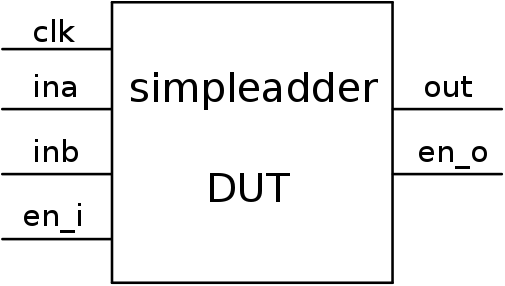# Chapter 1 – The DUT

This training guide will focus on showing how we can build a basic UVM environment, so the device under test was kept very simple in order to emphasize the explanation of UVM itself.

The DUT used is a simple ALU, limited to a single operation: the add operation. The inputs and outputs are represented in Figure 1.1.Figure 1.1: Representation of the DUT’s inputs/outputs

This DUT takes two values of 2 bits each, ina and inb, sums them and sends the result to the output out. The inputs are sampled to the signal of en_i and the output is sent at the same time en_o is signalled.

The operation of the DUT is represented as a timing diagram and as a state machine in Figure 1.2.

Figure 1.2: Operation of the DUT

The code for this DUT can be found here: